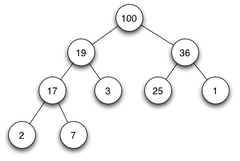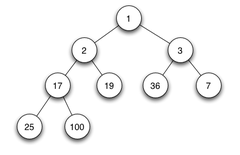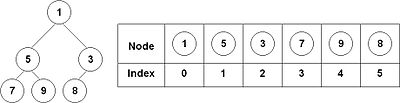# Binary heap

Binary heapExample of a complete binary max heapExample of a complete binary min heap

A binary heap is a heap data structure created using a binary tree. It can be seen as a binary tree with two additional constraints:

• The shape property: the tree is a complete binary tree; that is, all levels of the tree, except possibly the last one (deepest) are fully filled, and, if the last level of the tree is not complete, the nodes of that level are filled from left to right.
• The heap property: each node is greater than or equal to each of its children according to a comparison predicate defined for the data structure.

Heaps with a mathematical "greater than or equal to" comparison function are called max-heaps; those with a mathematical "less than or equal to" comparison function are called min-heaps. Min-heaps are often used to implement priority queues.

Since the ordering of siblings in a heap is not specified by the heap property, a single node's two children can be freely interchanged unless doing so violates the shape property (compare with treap).

The binary heap is a special case of the d-ary heap in which d = 2.

It is possible to modify the heap structure to allow extraction of both the smallest and largest element in O(log n) time. To do this, the rows alternate between min heap and max heap. The algorithms are roughly the same, but, in each step, one must consider the alternating rows with alternating comparisons. The performance is roughly the same as a normal single direction heap. This idea can be generalised to a min-max-median heap.

## Heap operations

### Insert

To add an element to a heap we must perform an up-heap operation (also known as bubble-up, percolate-up, sift-up, trickle up, heapify-up, or cascade-up) in order to restore the heap property. We can do this in O(log n) time, using a binary heap, by following this algorithm:

1. Add the element to the bottom level of the heap.
2. Compare the added element with its parent; if they are in the correct order, stop.
3. If not, swap the element with its parent and return to the previous step.

We do this at maximum once for each level in the tree—the height of the tree, which is O(log n). However, since approximately 50% of the elements are leaves and 75% are in the bottom two levels, it is likely that the new element to be inserted will only move a few levels upwards to maintain the heap. Thus, binary heaps support insertion in average constant time, O(1).

Say we have a max-heapand we want to add the number 15 to the heap. We first place the 15 in the position marked by the X. However, the heap property is violated since 15 is greater than 8, so we need to swap the 15 and the 8. So, we have the heap looking as follows after the first swap:However the heap property is still violated since 15 is greater than 11, so we need to swap again:which is a valid max-heap. There is no need to check the children after this. Before we placed 15 on X, the heap was valid, meaning 11 is greater than 5. If 15 is greater than 11, and 11 is greater than 5, then 15 must be greater than 5, because of the transitive relation.

### Remove

The procedure for deleting the root from the heap (effectively extracting the maximum element in a max-heap or the minimum element in a min-heap) and restoring the properties is called down-heap (also known as bubble-down, percolate-down, sift-down, trickle down, heapify-down, or cascade-down).

1. Replace the root of the heap with the last element on the last level.
2. Compare the new root with its children; if they are in the correct order, stop.
3. If not, swap the element with one of its children and return to the previous step. (Swap with its smaller child in a min-heap and its larger child in a max-heap.)

So, if we have the same max-heap as before, we remove the 11 and replace it with the 4.Now the heap property is violated since 8 is greater than 4. In this case, swapping the two elements 4 and 8, is enough to restore the heap property and we need not swap elements further:The downward-moving node is swapped with the larger of its children in a max-heap (in a min-heap it would be swapped with its smaller child), until it satisfies the heap property in its new position. This functionality is achieved by the Max-Heapify function as defined below in pseudocode for an array-backed heap A. Note that "A" is indexed starting at 1, not 0 as is common in many programming languages.

For the following algorithm to correctly re-heapify the array, the node at index i and its two direct children must violate the heap property. If they do not, the algorithm will fall through with no change to the array.

Max-Heapify(A, i):
left ← 2i
right ← 2i + 1
largesti
if leftheap_length[A] and A[left] > A[i] then:
largestleft
if rightheap_length[A] and A[right] > A[largest] then:
largestright
if largesti then:
swap A[i] ↔ A[largest]
Max-Heapify(A, largest)

The down-heap operation (without the preceding swap) can also be used to modify the value of the root, even when an element is not being deleted.

## Building a heap

A heap could be built by successive insertions. This approach requires O(nlog n) time because each insertion takes O(log n) time and there are n elements. However this is not the optimal method. The optimal method starts by arbitrarily putting the elements on a binary tree, respecting the shape property (the tree could be represented by an array, see below). Then starting from the lowest level and moving upwards, shift the root of each subtree downward as in the deletion algorithm until the heap property is restored. More specifically if all the subtrees starting at some height h (measured from the bottom) have already been "heapified", the trees at height h + 1 can be heapified by sending their root down along the path of maximum valued children when building a max-heap, or minimum valued children when building a min-heap. This process takes O(h) operations (swaps) per node. In this method most of the heapification takes place in the lower levels. The number of nodes at height h is$\le \left\lceil\frac{n}{2^{h+1}}\right\rceil$. Therefore, the cost of heapifying all subtrees is:\begin{align} \sum_{h=0}^{\lceil \lg n \rceil} \frac{n}{2^{h+1}}O(h) & = O\left(n\sum_{h=0}^{\lceil \lg n \rceil} \frac{h}{2^h}\right) \\ & \le O\left(n\sum_{h=0}^{\infty} \frac{h}{2^h}\right) \\ & = O(n) \end{align}

This uses the fact that the given infinite series h / 2h converges to 2.

The Build-Max-Heap function that follows, converts an array A which stores a complete binary tree with n nodes to a max-heap by repeatedly using Max-Heapify in a bottom up manner. It is based on the observation that the array elements indexed by floor(n/2) + 1, floor(n/2) + 2, ..., n are all leaves for the tree, thus each is a one-element heap. Build-Max-Heap runs Max-Heapify on each of the remaining tree nodes.

Build-Max-Heap(A):
heap_length[A] ← length[A]
for ifloor(length[A]/2) downto 1 do
Max-Heapify(A, i)

## Heap implementationComparison between a binary heap and an array implementation.

Heaps are commonly implemented with an array. Any binary tree can be stored in an array, but because a heap is always an almost complete binary tree, it can be stored compactly. No space is required for pointers; instead, the parent and children of each node can be found by arithmetic on array indices. These properties make this heap implementation a simple example of an implicit data structure or Ahnentafel list. Details depend on the root position, which in turn may depend on constraints of a programming language used for implementation, or programmer preference. Specifically, sometimes the root is placed at index 1, wasting space in order to simplify arithmetic.

Let n be the number of elements in the heap and i be an arbitrary valid index of the array storing the heap. If the tree root is at index 0, with valid indices 0 through n-1, then each element a[i] has

• children a[2i+1] and a[2i+2]
• parent a[floor((i−1)/2)]

Alternatively, if the tree root is at index 1, with valid indices 1 through n, then each element a[i] has

• children a[2i] and a[2i+1]
• parent a[floor(i/2)].

This implementation is used in the heapsort algorithm, where it allows the space in the input array to be reused to store the heap (i.e. the algorithm is done in-place). The implementation is also useful for use as a Priority queue where use of a dynamic array allows insertion of an unbounded number of items.

The upheap/downheap operations can then be stated in terms of an array as follows: suppose that the heap property holds for the indices b, b+1, ..., e. The sift-down function extends the heap property to b−1, b, b+1, ..., e. Only index i = b−1 can violate the heap property. Let j be the index of the largest child of a[i] (for a max-heap, or the smallest child for a min-heap) within the range b, ..., e. (If no such index exists because 2i > e then the heap property holds for the newly extended range and nothing needs to be done.) By swapping the values a[i] and a[j] the heap property for position i is established. At this point, the only problem is that the heap property might not hold for index j. The sift-down function is applied tail-recursively to index j until the heap property is established for all elements.

The sift-down function is fast. In each step it only needs two comparisons and one swap. The index value where it is working doubles in each iteration, so that at most log2 e steps are required.

For big heaps and using virtual memory, storing elements in an array according to the above scheme is inefficient: (almost) every level is in a different page. B-heaps are binary heaps that keep subtrees in a single page, reducing the number of pages accessed by up to a factor of ten.

The operation of merging two binary heaps takes Θ(n) for equal-sized heaps. The best you can do is (in case of array implementation) simply concatenating the two heap arrays and build a heap of the result. When merging is a common task, a different heap implementation is recommended, such as binomial heaps, which can be merged in O(log n).

Additionally, a binary heap can be implemented with a traditional binary tree data structure, but there is an issue with finding the adjacent element on the last level on the binary heap when adding an element. This element can be determined algorithmically or by adding extra data to the nodes, called "threading" the tree—instead of merely storing references to the children, we store the inorder successor of the node as well.

## Derivation of children's index in an array implementation

This derivation will show how for any given node i (starts from zero), its children would be found at 2i + 1 and 2i + 2.

### Mathematical proof

From the figure in "Heap Implementation" section, it can be seen that any node can store its children only after its right siblings and its left siblings' children have been stored. This fact will be used for derivation.

Total number of elements from root to any given level l = 2l + 1 − 1, where l starts at zero.

Suppose the node i is at level l.

So, the total number of nodes from root to previous level would be = 2(l − 1) + 1 − 1 = 2l − 1

Total number of nodes stored in the array till the index i = i + 1 (Counting i too)

So, total number of siblings on the left of i is

= Number of nodes including i − Number of nodes through the previous level − One node for i itself
= (i + 1) − (2l − 1) − 1
= i + 1 − 2l + 1 − 1
= i − 2l + 1

Hence, total number of children of these siblings = 2(i − 2l + 1)

Number of elements at any given level l = 2l

So, total siblings to right of i is:-

= Total nodes in level l − (Total siblings on left + 1)
= (2l) − (i − 2l + 2)
= 2l + 2li − 2)
= 2l + 1i − 2

So, index of 1st child of node i would be:-

= i + Total siblings on right + 2 * Total siblings on left + 1
= i + (2l + 1i − 2) + 2(i − 2l + 1) + 1
= i + 2l + 1i − 2 + 2i − 2l + 1 + 2 + 1
= ii + 2i + 2l + 1 − 2l + 1 − 2 + 2 + 1
= 2i + 1 [Proved]

### Intuitive proof

Although the mathematical approach proves this without doubt, but the simplicity of the resulting equation suggests that there should be a simpler way to arrive at this conclusion.

For this two facts should be noted.

• Children for node i will be found at the very first empty slot.
• Second is that, all nodes previous to node i, right from the root, will have exact two children. This is necessary to maintain the shape of the heap.

Now since all nodes have two children (as per the second fact) so all memory slots taken by the children will be 2((i + 1) − 1) = 2i. We add one since i starts at zero. Then we subtract one since node i doesn't yet have any children.

This means all filled memory slots have been accounted for except one – the root node. Root is child to none. So finally, the count of all filled memory slots are 2i + 1.

So, by fact one and since our indexing starts at zero, 2i + 1 itself gives the index of the first child of i.

Wikimedia Foundation. 2010.

### Look at other dictionaries:

• Heap (data structure) — This article is about the programming data structure. For the dynamic memory area, see Dynamic memory allocation. Example of a complete binary max heap In computer science, a heap is a specialized tree based data structure that satisfies the heap …   Wikipedia

• Binary tree — Not to be confused with B tree. A simple binary tree of size 9 and height 3, with a root node whose value is 2. The above tree is unbalanced and not sorted. In computer science, a binary tree is a tree data structure in which each node has at… …   Wikipedia

• Binary search tree — In computer science, a binary search tree (BST) is a binary tree data structurewhich has the following properties: *each node (item in the tree) has a value; *a total order (linear order) is defined on these values; *the left subtree of a node… …   Wikipedia

• Heap (mathematics) — In abstract algebra, a heap (sometimes also called a groud) is a mathematical generalisation of a group. Informally speaking, one obtains a heap from a group by forgetting which element is the unit, in the same way that one can think of an affine …   Wikipedia

• Binary Tree — Ein voller, aber nicht vollständiger Binärbaum Als Binärbaum bezeichnet man in der Graphentheorie eine spezielle Form eines Graphen. Genauer gesagt handelt es sich um einen gewurzelten Baum, bei dem jeder Knoten höchstens zwei Kindknoten besitzt …   Deutsch Wikipedia

• d-ary heap — The d ary heap or d heap is a priority queue data structure, a generalization of the binary heap in which the nodes have d children instead of 2. Thus, a binary heap is a 2 heap. According to Tarjan and Jensen et al …   Wikipedia

• Binomial heap — In computer science, a binomial heap is a heap similar to a binary heap but also supporting the operation of merging two heaps quickly. This is achieved by using a special tree structure. It is important as an implementation of the mergeable heap …   Wikipedia

• Min-max heap — A min max heap is a double ended priority queue implemented as a modified version of a binary heap. Like a binary heap, a min max heap is represented as a complete binary tree. Unlike a binary heap, though, the nodes in this tree do not obey the… …   Wikipedia

• Skew heap — A skew heap is a variant of a binary heap. In contrast to e.g. leftist heaps, there is no structural constraint on skew heaps.There are only two constraints left: * The general heap order must be enforced * Every operation (add, remove min,… …   Wikipedia

• Min heap order — means that the key in each node in a heap structure is less than the key(s) in all nodes that are children of that node of interest.See Binary heap for more details on heaps …   Wikipedia